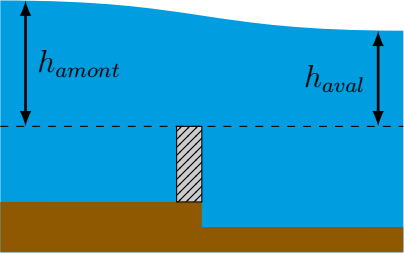# Submerged weir formulaExcerpt from: Rajaratnam, N., Muralidhar, D., 1969. Flow below deeply submerged rectangular weirs. Journal of Hydraulic Research 7, 355–374.

In submerged flow, the flow rate depends on the upstream water level $$h_{amont}$$ and the downstream water level $$h_{aval}$$ (Rajaratnam et al., 1969):

$Q = Cd \sqrt{2g} Lh_{aval} \sqrt{h_{amont}-h_{aval}}$

With:

• L the weir width in m
• hamont the upstream head on the weir in m
• haval the downstream head on the weir in m
• Cd the discharge coefficient (equal to 0.9 by default).

This formula is not recommended for flooding below 80%.

Rajaratnam, N., Muralidhar, D., 1969. Flow below deeply submerged rectangular weirs. Journal of Hydraulic Research 7, 355–374.